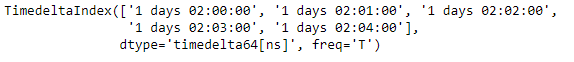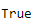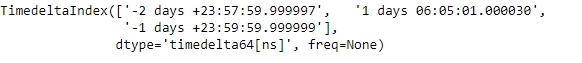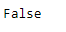# Python | Pandas TimedeltaIndex.is_monotonic_increasing

Python is a great language for doing data analysis, primarily because of the fantastic ecosystem of data-centric python packages. Pandas is one of those packages and makes importing and analyzing data much easier.

Pandas` TimedeltaIndex.is_monotonic_increasing` attribute return `True` if the index value are monotonically increasing. It return `True` even when the values are equal.

Syntax: TimedeltaIndex.is_monotonic_increasing

Return : boolean

Example #1: Use `TimedeltaIndex.is_monotonic_increasing` attribute to check if the TimedeltaIndex object is monotonic increasing or not.

 `# importing pandas as pd ` `import` `pandas as pd ` ` `  `# Create the TimedeltaIndex object ` `tidx ``=` `pd.TimedeltaIndex(start ``=``'1 days 02:00:00'``, periods ``=` `5``, freq ``=``'T'``) ` ` `  `# Print the TimedeltaIndex ` `print``(tidx) `

Output :Now we will check if the TimedeltaIndex object is monotonic increasing or not.

 `# check if tidx is monotonic increasing or not ` `tidx.is_monotonic_increasing `

Output :As we can see in the output, the `TimedeltaIndex.is_monotonic_increasing` attribute has returned `True` indicating that value in tidx object is monotonically increasing.

Example #2: Use `TimedeltaIndex.is_monotonic_increasing` attribute to check if the TimedeltaIndex object is monotonic increasing or not.

 `# importing pandas as pd ` `import` `pandas as pd ` ` `  `# Create the TimedeltaIndex object ` `tidx ``=` `pd.TimedeltaIndex(data ``=``[``'-1 days 2 min 3us'``, ``'1 days 06:05:01.000030'``, ` `                                                ``'-1 days + 23:59:59.999999'``]) ` ` `  `# Print the TimedeltaIndex ` `print``(tidx) `

Output :Now we will check if the TimedeltaIndex object is monotonic increasing or not.

 `# check if tidx is monotonic increasing or not ` `tidx.is_monotonic_increasing `

Output :As we can see in the output, the `TimedeltaIndex.is_monotonic_increasing` attribute has returned `False` indicating that value in tidx object is not monotonically increasing.

My Personal Notes arrow_drop_upCheck out this Author's contributed articles.

If you like GeeksforGeeks and would like to contribute, you can also write an article using contribute.geeksforgeeks.org or mail your article to contribute@geeksforgeeks.org. See your article appearing on the GeeksforGeeks main page and help other Geeks.

Please Improve this article if you find anything incorrect by clicking on the "Improve Article" button below.

Article Tags :

Be the First to upvote.

Please write to us at contribute@geeksforgeeks.org to report any issue with the above content.